# fan2para

Convert fan-beam projections to parallel-beam

## Syntax

P = fan2para(F,D)
P = fan2para(F,D,Name,Value)
[P,paraSensorPos,paraRotAngles] = fan2para(___)

## Description

P = fan2para(F,D) converts the fan-beam data F to the parallel-beam data P. Each column of F contains the fan-beam data at one rotation angle. D is the distance from the fan-beam vertex to the center of rotation.

example

P = fan2para(F,D,Name,Value) uses name-value arguments to control aspects of the data conversion.
[P,paraSensorPos,paraRotAngles] = fan2para(___) returns the parallel-beam sensor locations in paraSensorPos and rotation angles in paraRotAngles.

## Examples

collapse all

Create synthetic parallel-beam data.

ph = phantom(128);

Calculate the parallel beam transform and display it.

theta = 0:179; [Psynthetic,xp] = radon(ph,theta); imshow(Psynthetic,[],... 'XData',theta,'YData',xp,'InitialMagnification','fit') axis normal title('Synthetic Parallel-Beam Data') xlabel('\theta (degrees)') ylabel('x''') colormap(gca,hot), colorbar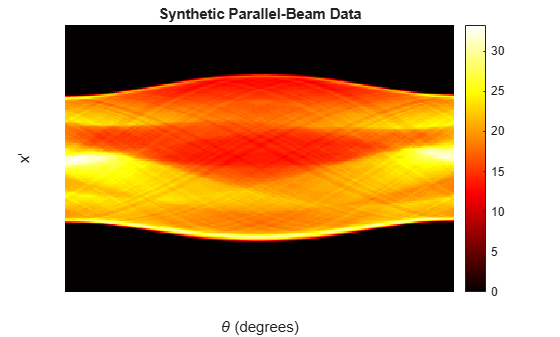Convert the parallel-beam data to fan-beam.

Fsynthetic = para2fan(Psynthetic,100,'FanSensorSpacing',1);

Recover original parallel-beam data.

[Precovered,Ploc,Pangles] = fan2para(Fsynthetic,100,... 'FanSensorSpacing',1,... 'ParallelSensorSpacing',1); figure imshow(Precovered,[],... 'XData',Pangles,'YData',Ploc,'InitialMagnification','fit') axis normal title('Recovered Parallel-Beam Data') xlabel('Rotation Angles (degrees)') ylabel('Parallel Sensor Locations (pixels)') colormap(gca,hot), colorbar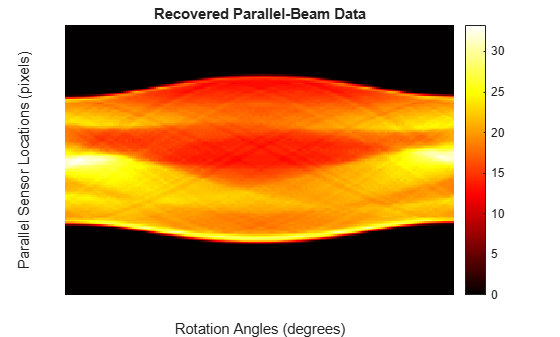## Input Arguments

collapse all

Fan-beam projection data, specified as a numeric matrix. Each column of F contains the fan-beam data at one rotation angle. The number of columns indicates the number of fan-beam rotation angles and the number of rows indicates the number of fan-beam sensors.

Data Types: double | single

Distance in pixels from the fan beam vertex to the center of rotation, specified as a positive number. fan2para assumes that the center of rotation is the center point of the projections, which is defined as ceil(size(F,1)/2). The figure illustrates D in relation to the fan-beam vertex for one fan-beam projection.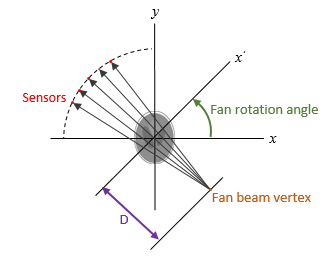Data Types: double | single

### Name-Value Arguments

Specify optional pairs of arguments as Name1=Value1,...,NameN=ValueN, where Name is the argument name and Value is the corresponding value. Name-value arguments must appear after other arguments, but the order of the pairs does not matter.

Example: P = fan2para(F,D,FanRotationIncrement=5) specifies a fan rotation increment of 5 degrees.

Before R2021a, use commas to separate each name and value, and enclose Name in quotes.

Example: P = fan2para(F,D,"FanRotationIncrement",5) specifies a fan rotation increment of 5 degrees.

Range of fan-beam rotation, specified as "cycle" or "minimal".

• "cycle" — Rotate through the full range [0, 360) degrees.

• "minimal" — Rotate through the minimum range necessary to represent the object.

Fan-beam rotation angle increment in degrees, specified as a positive scalar.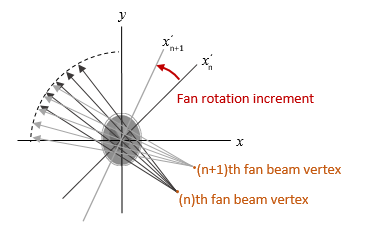Data Types: double

Fan-beam sensor positioning, specified as "arc" or "line".

Value

Meaning

Diagram

"arc"

Sensors are spaced at equal angles along a circular arc. The center of the arc is the fan-beam vertex.

FanSensorSpacing defines the angular spacing in degrees.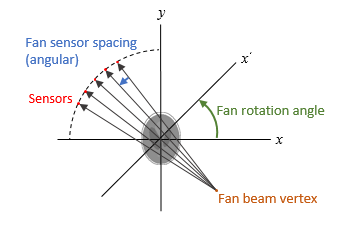"line"

Sensors are spaced at equal distances along a line that is parallel to the x' axis. The closest sensor is distance D from the center of rotation.

FanSensorSpacing defines the distance between fan-beams on the x' axis, in pixels.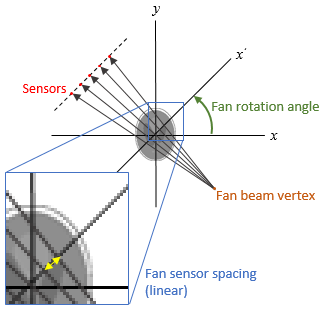Fan-bean sensor spacing, specified as a positive scalar.

• If FanSensorGeometry is "arc", then FanSensorSpacing defines the angular spacing in degrees.

• If FanSensorGeometry is "line", then FanSensorSpacing defines the linear distance between fan-beams, in pixels. Linear spacing is measured on the x' axis.

Data Types: double

Type of interpolation used between the parallel-beam and fan-beam data, specified as one of these values.

"nearest" — Nearest-neighbor

"linear" — Linear (the default)

"spline" — Piecewise cubic spline

"pchip" — Piecewise cubic Hermite (PCHIP)

Range of parallel-beam rotation, specified as "halfcycle" or "cycle".

• "cycle" — Parallel data covers the full range of [0, 360) degrees.

• "halfcycle" — Parallel data covers [0, 180) degrees.

Parallel-beam rotation angle increment in degrees, specified as a positive scalar k such that 180/k is an integer. If you do not specify ParallelRotationIncrement, then the default value is equal to FanRotationIncrement.

Data Types: double

Parallel-beam sensor spacing in pixels, specified as a positive scalar. The range of parallel-beam sensor locations is computed from the range of fan angles, fanAngles, according to: [D*sin(min(fanAngles)) D*sin(max(fanAngles))].

If you do not specify ParallelSensorSpacing, then the spacing is assumed to be uniform and is set to the minimum spacing implied by the fan angles and sampled over the range implied by the fan angles.

Data Types: double

## Output Arguments

collapse all

Parallel-beam projection data, returned as a numeric matrix. Each column of P contains the parallel-beam data at one rotation angle. The number of columns indicates the total number of parallel-beam rotation angles and is equal to the length of paraRotAngles. The number of rows indicates the total number of parallel-beam sensors and is equal to the length of paraSensorPos.

Data Types: double

Parallel-beam sensor locations, returned as a numeric column vector.

Data Types: double

Parallel-beam rotation angles, returned as a numeric row vector.

Data Types: double

## Version History

Introduced before R2006a